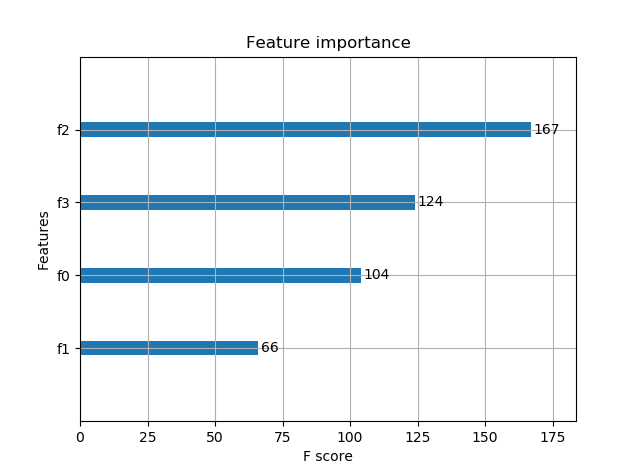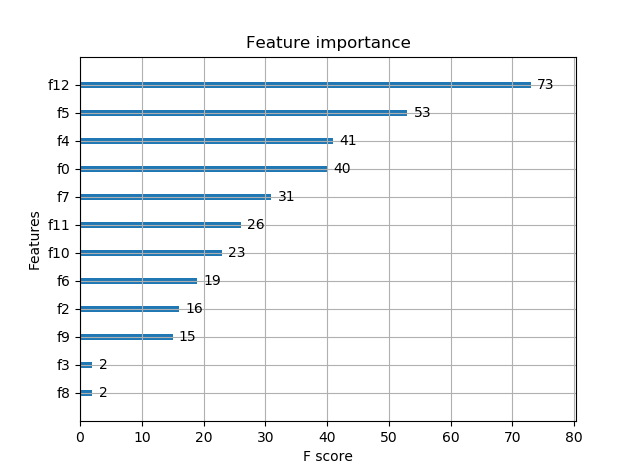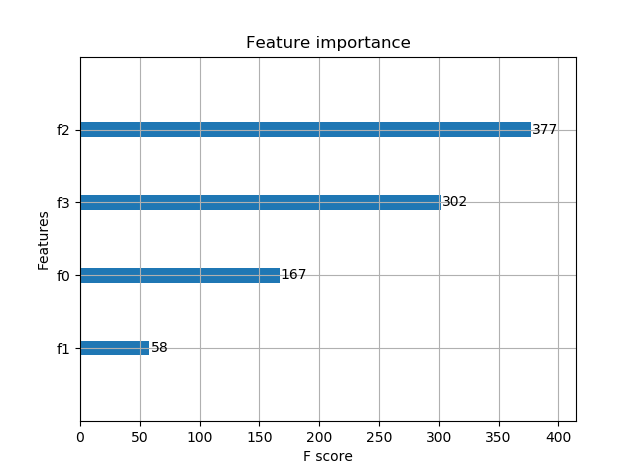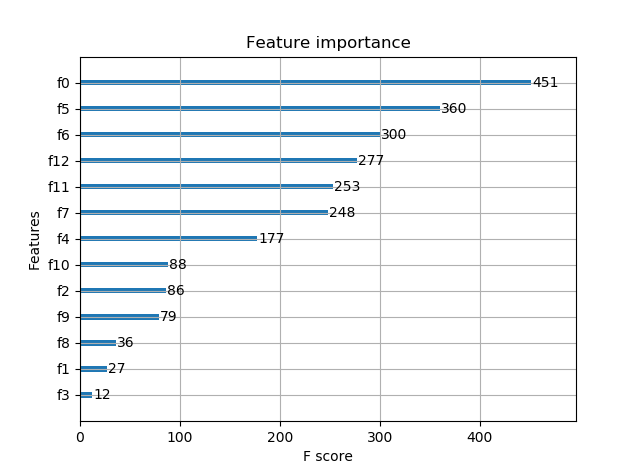# python机器学习库xgboost——xgboost算法

python数据挖掘系列教程

## 安装

xgboost目前还不能pip在线安装，所以先在网址https://www.lfd.uci.edu/~gohlke/pythonlibs/#xgboost 中下载whl文件，然后参考https://blog.csdn.net/luanpeng825485697/article/details/77816740 进行离线安装，就可以正常导入xgboost库了。

## xgboost简介

xgboost一般和sklearn一起使用，但是由于sklearn中没有集成xgboost，所以才需要单独下载安装。

xgboost是在GBDT的基础上进行改进，使之更强大，适用于更大范围。

## 2. XGBoost的优点

2.1 正则化

XGBoost在代价函数里加入了正则项，用于控制模型的复杂度。正则项里包含了树的叶子节点个数、每个叶子节点上输出的score的L2模的平方和。从Bias-variance tradeoff角度来讲，正则项降低了模型的variance，使学习出来的模型更加简单，防止过拟合，这也是xgboost优于传统GBDT的一个特性。

2.2 并行处理

XGBoost工具支持并行。Boosting不是一种串行的结构吗?怎么并行的？注意XGBoost的并行不是tree粒度的并行，XGBoost也是一次迭代完才能进行下一次迭代的（第t次迭代的代价函数里包含了前面t-1次迭代的预测值）。XGBoost的并行是在特征粒度上的。

我们知道，决策树的学习最耗时的一个步骤就是对特征的值进行排序（因为要确定最佳分割点），XGBoost在训练之前，预先对数据进行了排序，然后保存为block结构，后面的迭代中重复地使用这个结构，大大减小计算量。这个block结构也使得并行成为了可能，在进行节点的分裂时，需要计算每个特征的增益，最终选增益最大的那个特征去做分裂，那么各个特征的增益计算就可以开多线程进行。

2.3 灵活性

XGBoost支持用户自定义目标函数和评估函数，只要目标函数二阶可导就行。

2.4 缺失值处理

对于特征的值有缺失的样本，xgboost可以自动学习出它的分裂方向

2.5 剪枝

XGBoost 先从顶到底建立所有可以建立的子树，再从底到顶反向进行剪枝。比起GBM，这样不容易陷入局部最优解。

2.6 内置交叉验证

XGBoost允许在每一轮boosting迭代中使用交叉验证。因此，可以方便地获得最优boosting迭代次数。而GBM使用网格搜索，只能检测有限个值。

## 3. XGBoost详解

3.1 数据格式

XGBoost可以加载多种数据格式的训练数据：

libsvm 格式的文本数据；

Numpy 的二维数组；

XGBoost 的二进制的缓存文件。加载的数据存储在对象 DMatrix 中。

 dtrain1 = xgb.DMatrix('train.svm.txt')

dtrain2 = xgb.DMatrix('train.svm.buffer')

data = np.random.rand(5,10) # 5行10列数据集
label = np.random.randint(2, size=5) # 2分类目标值
dtrain = xgb.DMatrix( data, label=label) # 组成训练集

csr = scipy.sparse.csr_matrix( (dat, (row,col)) )
dtrain = xgb.DMatrix( csr )

 dtrain = xgb.DMatrix('train.svm.txt')
dtrain.save_binary("train.buffer")

dtrain = xgb.DMatrix( data, label=label, missing = -999.0)

w = np.random.rand(5,1)
dtrain = xgb.DMatrix( data, label=label, missing = -999.0, weight=w)

3.2 参数设置

XGBoost使用key-value字典的方式存储参数：

params = {
'booster': 'gbtree',
'objective': 'multi:softmax',  # 多分类的问题
'num_class': 10,               # 类别数，与 multisoftmax 并用
'gamma': 0.1,                  # 用于控制是否后剪枝的参数,越大越保守，一般0.1、0.2这样子。
'max_depth': 12,               # 构建树的深度，越大越容易过拟合
'lambda': 2,                   # 控制模型复杂度的权重值的L2正则化项参数，参数越大，模型越不容易过拟合。
'subsample': 0.7,              # 随机采样训练样本
'colsample_bytree': 0.7,       # 生成树时进行的列采样
'min_child_weight': 3,
'silent': 1,                   # 设置成1则没有运行信息输出，最好是设置为0.
'eta': 0.007,                  # 如同学习率
'seed': 1000,
'nthread': 4,                  # cpu 线程数
}

3.3 训练模型

num_round = 10
bst = xgb.train( plst, dtrain, num_round, evallist )

3.4 模型预测

# X_test类型可以是二维List，也可以是numpy的数组
dtest = DMatrix(X_test)
ans = model.predict(dtest)

3.5 保存模型

 bst.save_model('test.model')

# 导出模型到文件
bst.dump_model('dump.raw.txt')
# 导出模型和特征映射
bst.dump_model('dump.raw.txt','featmap.txt')

3.6 加载模型

bst = xgb.Booster({'nthread':4}) # init model
bst.load_model("model.bin")      # load data

## XGBoost参数详解

在运行XGboost之前，必须设置三种类型成熟：general parameters，booster parameters和task parameters：

Booster参数 ：这取决于使用哪种booster。

## 4.1 通用参数

booster [default=gbtree]：有两中模型可以选择gbtree和gblinear。gbtree使用基于树的模型进行提升计算，gblinear使用线性模型进行提升计算。缺省值为gbtree

silent [default=0]：取0时表示打印出运行时信息，取1时表示以缄默方式运行，不打印运行时信息。缺省值为0

nthread：XGBoost运行时的线程数。缺省值是当前系统可以获得的最大线程数

num_pbuffer：预测缓冲区大小，通常设置为训练实例的数目。缓冲用于保存最后一步提升的预测结果，无需人为设置。

num_feature：Boosting过程中用到的特征维数，设置为特征个数。XGBoost会自动设置，无需人为设置。

## 4.2 tree booster参数

eta [default=0.3] ：为了防止过拟合，更新过程中用到的收缩步长。在每次提升计算之后，算法会直接获得新特征的权重。 eta通过缩减特征的权重使提升计算过程更加保守。缺省值为0.3

gamma [default=0] ：在节点分裂时，只有分裂后损失函数的值下降了，才会分裂这个节点。Gamma指定了节点分裂所需的最小损失函数下降值。

max_depth [default=6] ：数的最大深度。缺省值为6 。取值范围为：[1,∞]。需要使用CV函数来进行调优。典型值：3-10

min_child_weight [default=1] ：孩子节点中最小的样本权重和。如果一个叶子节点的样本权重和小于min_child_weight则拆分过程结束。在现行回归模型中，这个参数是指建立每个模型所需要的最小样本数。这个参数用于避免过拟合。当它的值较大时，可以避免模型学习到局部的特殊样本。

max_delta_step [default=0] ：我们允许每个树的权重被估计的值。如果它的值被设置为0，意味着没有约束；如果它被设置为一个正值，它能够使得更新的步骤更加保守。通常这个参数是没有必要的，但是如果在逻辑回归中类极其不平衡这时候他有可能会起到帮助作用。把它范围设置为1-10之间也许能控制更新。 取值范围为：[0,∞]

subsample [default=1] ：用于训练模型的子样本占整个样本集合的比例。如果设置为0.5则意味着XGBoost将随机的从整个样本集合中随机的抽取出50%的子样本建立树模型，这能够防止过拟合。 取值范围为：(0,1]

colsample_bytree [default=1] ：在建立树时对特征采样的比例。缺省值为1 。取值范围为：(0,1]

## 4.3 Linear Booster参数

lambda [default=0] ：L2 正则的惩罚系数

alpha [default=0] ：L1 正则的惩罚系数

lambda_bias ：在偏置上的L2正则。缺省值为0（在L1上没有偏置项的正则，因为L1时偏置不重要）

## 4.4 学习目标参数

objective [ default=reg:linear ] ：定义学习任务及相应的学习目标，可选的目标函数如下：

• “reg:linear” —— 线性回归。
• “reg:logistic”—— 逻辑回归。
• “binary:logistic”—— 二分类的逻辑回归问题，输出为概率。
• “binary:logitraw”—— 二分类的逻辑回归问题，输出的结果为wTx。
• “count:poisson”—— 计数问题的poisson回归，输出结果为poisson分布。在poisson回归中，max_delta_step的缺省值为0.7。(used to safeguard optimization)
• “multi:softmax” –让XGBoost采用softmax目标函数处理多分类问题，同时需要设置参数num_class（类别个数）
• “multi:softprob” –和softmax一样，但是输出的是ndata * nclass的向量，可以将该向量reshape成ndata行nclass列的矩阵。没行数据表示样本所属于每个类别的概率。
• “rank:pairwise” –set XGBoost to do ranking task by minimizing the pairwise loss

base_score [ default=0.5 ]

eval_metric [ default according to objective ]

• rmse 均方根误差($\sqrt{\frac{\sum _{i=1}^{N}{ϵ}^{2}}{N}}$$\sqrt {\frac{\sum_{i=1}^Nϵ^2}{N}}$)
• mae 平均绝对误差($\frac{\sum _{i=1}^{N}|ϵ|}{N}$$\frac{\sum_{i=1}^N|ϵ|}{N}$)
• logloss 负对数似然函数值
• error 二分类错误率(阈值为0.5)
• merror 多分类错误率
• mlogloss 多分类logloss损失函数
• auc 曲线下面积

seed [ default=0 ]

## xgboost 基本方法和默认参数

xgboost.train(params,dtrain,num_boost_round=10,evals=(),obj=None,feval=None,maximize=False,early_stopping_rounds=None,evals_result=None,verbose_eval=True,learning_rates=None,xgb_model=None)  

params ：这是一个字典，里面包含着训练中的参数关键字和对应的值，形式是params = {‘booster’:’gbtree’, ’eta’:0.1}
dtrain ：训练的数据
num_boost_round ：这是指提升迭代的个数
evals ：这是一个列表，用于对训练过程中进行评估列表中的元素。形式是evals = [(dtrain,’train’), (dval,’val’)]或者是evals = [ (dtrain,’train’)], 对于第一种情况，它使得我们可以在训练过程中观察验证集的效果。
obj：自定义目的函数
feval：自定义评估函数
maximize： 是否对评估函数进行最大化
early_stopping_rounds： 早期停止次数 ，假设为100，验证集的误差迭代到一定程度在100次内不能再继续降低，就停止迭代。这要求evals 里至少有一个元素，如果有多个，按最后一个去执行。返回的是最后的迭代次数（不是最好的）。如果early_stopping_rounds 存在，则模型会生成三个属性，bst.best_score, bst.best_iteration, 和bst.best_ntree_limit
evals_result ：字典，存储在watchlist 中的元素的评估结果。
verbose_eval(可以输入布尔型或数值型)：也要求evals 里至少有 一个元素。如果为True, 则对evals中元素的评估结果会输出在结果中；如果输入数字，假设为5，则每隔5个迭代输出一次。
learning_rates ：每一次提升的学习率的列表，
xgb_model：在训练之前用于加载的xgb model。

## 5. XGBoost实战

XGBoost有两大类接口：XGBoost原生接口 和 scikit-learn接口 ，并且XGBoost能够实现 分类 和 回归 两种任务。因此，本章节分四个小块来介绍！

5.1 基于XGBoost原生接口的分类

# ================基于XGBoost原生接口的分类=============
from sklearn.datasets import load_iris
import xgboost as xgb
from xgboost import plot_importance
from matplotlib import pyplot as plt
from sklearn.model_selection import train_test_split
from sklearn.metrics import accuracy_score   # 准确率
# 加载样本数据集
iris = load_iris()
X,y = iris.data,iris.target
X_train, X_test, y_train, y_test = train_test_split(X, y, test_size=0.2, random_state=1234565) # 数据集分割

# 算法参数
params = {
'booster': 'gbtree',
'objective': 'multi:softmax',
'num_class': 3,
'gamma': 0.1,
'max_depth': 6,
'lambda': 2,
'subsample': 0.7,
'colsample_bytree': 0.7,
'min_child_weight': 3,
'silent': 1,
'eta': 0.1,
'seed': 1000,
'nthread': 4,
}

plst = params.items()

dtrain = xgb.DMatrix(X_train, y_train) # 生成数据集格式
num_rounds = 500
model = xgb.train(plst, dtrain, num_rounds) # xgboost模型训练

# 对测试集进行预测
dtest = xgb.DMatrix(X_test)
y_pred = model.predict(dtest)

# 计算准确率
accuracy = accuracy_score(y_test,y_pred)
print("accuarcy: %.2f%%" % (accuracy*100.0))

# 显示重要特征
plot_importance(model)
plt.show()

Accuracy: 96.67 %5.2 基于XGBoost原生接口的回归

# ================基于XGBoost原生接口的回归=============

import xgboost as xgb
from xgboost import plot_importance
from matplotlib import pyplot as plt
from sklearn.model_selection import train_test_split
from sklearn.datasets import load_boston
from sklearn.metrics import mean_squared_error

# 加载数据集
boston = load_boston()
X,y = boston.data,boston.target

# XGBoost训练过程
X_train, X_test, y_train, y_test = train_test_split(X, y, test_size=0.2, random_state=0)

params = {
'booster': 'gbtree',
'objective': 'reg:gamma',
'gamma': 0.1,
'max_depth': 5,
'lambda': 3,
'subsample': 0.7,
'colsample_bytree': 0.7,
'min_child_weight': 3,
'silent': 1,
'eta': 0.1,
'seed': 1000,
'nthread': 4,
}

dtrain = xgb.DMatrix(X_train, y_train)
num_rounds = 300
plst = params.items()
model = xgb.train(plst, dtrain, num_rounds)

# 对测试集进行预测
dtest = xgb.DMatrix(X_test)
ans = model.predict(dtest)

# 显示重要特征
plot_importance(model)
plt.show()## xgb使用sklearn接口(推荐)

XGBClassifier

from xgboost.sklearn import XGBClassifier
clf = XGBClassifier(
silent=0 ,#设置成1则没有运行信息输出，最好是设置为0.是否在运行升级时打印消息。
#nthread=4,# cpu 线程数 默认最大
learning_rate= 0.3, # 如同学习率
min_child_weight=1,
# 这个参数默认是 1，是每个叶子里面 h 的和至少是多少，对正负样本不均衡时的 0-1 分类而言
#，假设 h 在 0.01 附近，min_child_weight 为 1 意味着叶子节点中最少需要包含 100 个样本。
#这个参数非常影响结果，控制叶子节点中二阶导的和的最小值，该参数值越小，越容易 overfitting。
max_depth=6, # 构建树的深度，越大越容易过拟合
gamma=0,  # 树的叶子节点上作进一步分区所需的最小损失减少,越大越保守，一般0.1、0.2这样子。
subsample=1, # 随机采样训练样本 训练实例的子采样比
max_delta_step=0,#最大增量步长，我们允许每个树的权重估计。
colsample_bytree=1, # 生成树时进行的列采样
reg_lambda=1,  # 控制模型复杂度的权重值的L2正则化项参数，参数越大，模型越不容易过拟合。
#reg_alpha=0, # L1 正则项参数
#scale_pos_weight=1, #如果取值大于0的话，在类别样本不平衡的情况下有助于快速收敛。平衡正负权重
#objective= 'multi:softmax', #多分类的问题 指定学习任务和相应的学习目标
#num_class=10, # 类别数，多分类与 multisoftmax 并用
n_estimators=100, #树的个数
seed=1000 #随机种子
#eval_metric= 'auc'
)
clf.fit(X_train,y_train,eval_metric='auc')

5.3 基于Scikit-learn接口的分类

# ==============基于Scikit-learn接口的分类================
from sklearn.datasets import load_iris
import xgboost as xgb
from xgboost import plot_importance
from matplotlib import pyplot as plt
from sklearn.model_selection import train_test_split
from sklearn.metrics import accuracy_score

# 加载样本数据集
iris = load_iris()
X,y = iris.data,iris.target
X_train, X_test, y_train, y_test = train_test_split(X, y, test_size=0.2, random_state=1234565) # 数据集分割

# 训练模型
model = xgb.XGBClassifier(max_depth=5, learning_rate=0.1, n_estimators=160, silent=True, objective='multi:softmax')
model.fit(X_train, y_train)

# 对测试集进行预测
y_pred = model.predict(X_test)

# 计算准确率
accuracy = accuracy_score(y_test,y_pred)
print("accuarcy: %.2f%%" % (accuracy*100.0))

# 显示重要特征
plot_importance(model)
plt.show()# ================基于Scikit-learn接口的回归================
import xgboost as xgb
from xgboost import plot_importance
from matplotlib import pyplot as plt
from sklearn.model_selection import train_test_split
from sklearn.datasets import load_boston

boston = load_boston()
X,y = boston.data,boston.target

# XGBoost训练过程
X_train, X_test, y_train, y_test = train_test_split(X, y, test_size=0.2, random_state=0)

model = xgb.XGBRegressor(max_depth=5, learning_rate=0.1, n_estimators=160, silent=True, objective='reg:gamma')
model.fit(X_train, y_train)

# 对测试集进行预测
ans = model.predict(X_test)

# 显示重要特征
plot_importance(model)
plt.show()## 参数调优的一般方法

1. 选择较高的学习速率(learning rate)。一般情况下，学习速率的值为0.1。但是，对于不同的问题，理想的学习速率有时候会在0.05到0.3之间波动。选择对应于此学习速率的理想决策树数量。XGBoost有一个很有用的函数“cv”，这个函数可以在每一次迭代中使用交叉验证，并返回理想的决策树数量。
2. 对于给定的学习速率和决策树数量，进行决策树特定参数调优(max_depth, min_child_weight, gamma, subsample, colsample_bytree)。在确定一棵树的过程中，我们可以选择不同的参数，待会儿我会举例说明。
3. xgboost的正则化参数的调优。(lambda, alpha)。这些参数可以降低模型的复杂度，从而提高模型的表现。
4. 降低学习速率，确定理想参数。

1、max_depth = 5 :这个参数的取值最好在3-10之间。我选的起始值为5，但是你也可以选择其它的值。起始值在4-6之间都是不错的选择。
2、min_child_weight = 1:在这里选了一个比较小的值，因为这是一个极不平衡的分类问题。因此，某些叶子节点下的值会比较小。
3、gamma = 0: 起始值也可以选其它比较小的值，在0.1到0.2之间就可以。这个参数后继也是要调整的。
4、subsample,colsample_bytree = 0.8: 这个是最常见的初始值了。典型值的范围在0.5-0.9之间。
5、scale_pos_weight = 1: 这个值是因为类别十分不平衡。